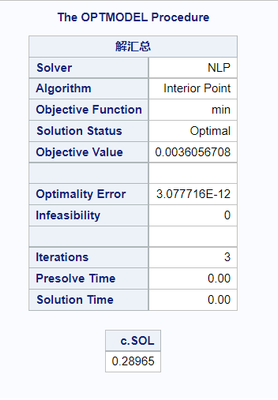## get a value after minimizing sum of squares

data have1;

input bucket\$  probability ;
cards;
first  0.0025
second  0.37
third  0.53
fourth  0.89
;
run;

data have2;

input bucket\$  z;
cards;
first -2.81
second -0.60
third -0.15
fourth 0.69

;
run;

Hi Everyone,

I have 2 data sets as above. I want to calculate a value(v) as

v = cdf('normal', z+c)

where z = z-value from table have2

c = constant which we will start with 1 and continuously changing it to get the minimum sum of squares difference of V and probability( from table have1).

We are interested in getting the value of C (initially equals 1) such that (V - probability)^2 is minimised.

5 REPLIES 5Ksharp
Super User

## Re: get a value after minimizing sum of squares

If you have SAS/OR ,could try the following code . or calling @RobPratt

Or if you have SAS/IML ,could try many optimize function like CALL NLPNRA () ,CALL NLPLM() .

@Rick_SAS  wrote may blogs about it.

``````data have1;
input bucket\$  probability ;
cards;
first  0.0025
second  0.37
third  0.53
fourth  0.89
;
run;

data have2;
input bucket\$  z;
cards;
first -2.81
second -0.60
third -0.15
fourth 0.69

;
run;

proc optmodel;
set <str> B;
num p{B} ;
num z{B};
read data have1 into B=[bucket] p=probability;
read data have2 into B=[bucket] z=z;

var c ;

min min=sum{i in B} (cdf('normal', z[i]+c)-p[i])**2;
solve ;

print c.sol;
quit;``````## Re: get a value after minimizing sum of squares

Hi, Thank you for your quick response. I don't have the mentioned components. Can we use SAS/STAT in this. Or is there any other other way without using SAS/OR and SAS/IML. Thanks.

## Re: get a value after minimizing sum of squares

Looks correct to me.  I have only two suggestions.

1. Change the second READ DATA statement as follows:

``read data have2 into [bucket] z;``

The index set B has already been populated from the previous READ DATA statement, and z=z is redundant.

2. Use the INIT option to initialize c, as requested by the user:

``````   var c init 1;
``````

## Re: get a value after minimizing sum of squares

Hi RobPratt,
Can we do this without using SAS/OR and SAS/IML? I don't have the required components as of now.
Can we use SAS/STAT in any way for this?Ksharp
Super User

## Re: get a value after minimizing sum of squares

OK. Here is. The same result with SAS/OR .

``````data have1;
input bucket\$  probability ;
cards;
first  0.0025
second  0.37
third  0.53
fourth  0.89
;
run;
proc sort data=have1;by bucket;run;

data have2;
input bucket\$  z;
cards;
first -2.81
second -0.60
third -0.15
fourth 0.69

;
run;
proc sort data=have2;by bucket;run;

data want;
merge have1 have2;
by bucket;
run;

proc nlin data=want;
parms c 1;
mean=cdf('normal', z+c);

model probability=mean;
run;``````
Discussion stats
• 5 replies
• 394 views
• 3 likes
• 3 in conversation